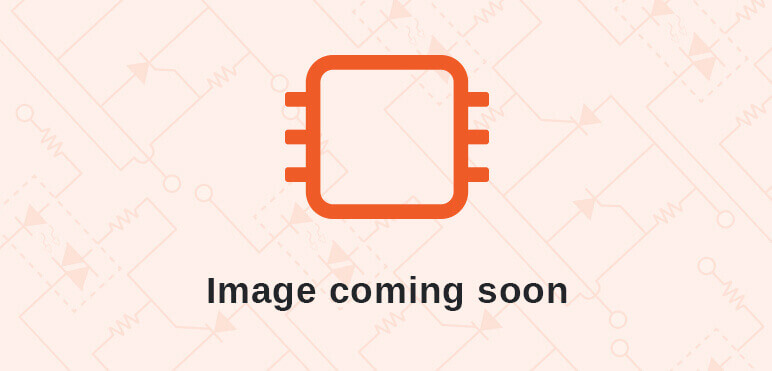# Signed Unsigned Multiplier and Divider Test Circuits### Details

Category: Arithmetic Core

Created: April 07, 2018

Updated: January 27, 2020

Language: VHDL

### Other project properties

Development Status: Beta

Additional info: Design done, FPGA proven

WishBone compliant: No

WishBone version: n/a

## Description

Goal:

Implement signed/unsigned 32/16 bit multiplier/divider using a finite state machine (and use it for a fun project)

Background:

I needed a signed / unsigned multiplier / divider for a different project, and decided to use algorithms described in this book: https://www.amazon.com/Computer-Organization-Architecture-Principles-Structure/dp0024154954

The interface is self-explanatory and should be easy enough to use in various projects:

entity signedmultiplier is
Port ( reset : in STD_LOGIC; -- Active high to initialize. Note that ready will be low as long as reset remains high
clk : in STD_LOGIC; -- Clock to drive the FSM
start : in STD_LOGIC; -- Apply high for at least 1 clock cycle to start computation
mode: in STD_LOGIC_VECTOR(1 downto 0);-- 00: unsigned multiply, 01: signed multiply, 10: unsigned divide, 11: signed divide
dividend32: in STD_LOGIC; -- 0: 16/16 divide, 1: 32/16 divide. ignored for multiplication
arg0h : in STD_LOGIC_VECTOR (15 downto 0); -- used as MSW for 32/16 divide
arg0l : in STD_LOGIC_VECTOR (15 downto 0); -- LSW for 32/16 divide, factor0 for 16*16 multiplication
arg1 : in STD_LOGIC_VECTOR (15 downto 0); -- factor1 for 16*16 multiplication
result : buffer STD_LOGIC_VECTOR (31 downto 0); -- 32 bit result when multiplying, when dividing quotient is MSW, remainder is LSW
ready : out STD_LOGIC; -- goes high when done
error : out STD_LOGIC; -- goes high when dividing by 0
zero : out STD_LOGIC; -- quotient is zero (divide) or whole product is zero (multiplication)
sign : out STD_LOGIC; -- same result checked like for zero, only meaningful for signed operation
debug : out STD_LOGIC_VECTOR(3 downto 0)); -- debug output (leave open)
end signedmultiplier;

The 16-bit multiply / divide modes have been tested pretty thoroughly, but the 32/16 may still have some bugs...

Debugging:

Very simple hardware debugging was used - a single step circuit combined with key signals from the guts of the components, displaying the data on Mercury baseboard 7seg LEDs and using buttons and switches there.

Testing:

To test the functionality of the multiplier / dividers, 3 different approaches can be used:

---------------------------------------------------------------------------------------------------
SW(4) SW(0) Circuit Input Output
---------------------------------------------------------------------------------------------------
0 0 Manual "calculator" (use SW(3:0) to select operation) PMOD Keyboard 4 7-seg LED
0 1 - " - UART UART
1 0 Prime number generator N/A N/A
1 1 - " - UART UART
---------------------------------------------------------------------------------------------------

The I/O can be thought of as 2 input (BTN(1) pressed) registers that are "shifted up" by 1 hex digit any time PMOD keyboard is pressed, or a character is received through UART. When BTN(1) is released, result MSW or LSW are displayed on the LEDs.

Prime number generator:

The algorithm used is described in "findprimes.bs2" Basic Stamp program. It uses unsigned multiplication and division (checking for remainder) to determine if the integer is prime or not. It runs on a "mini CPU" which is a simple data path processor driven by microcode which encodes the prime finding algorithm. When a prime number is found, its order and value are sent through UART (57600 baud, 8-N-1)

Here is one example of operation:

1. Switches: 01111XXX1 = no single step, 25MHz, prime generator, UART use
2. Press button 3 to start clock
3. Press buttons 1 to display lower boundary and enter as hex through serial terminal (e.g. 0000)
4. Press buttons 1 and 0 to display upper boundary and enter as hex through serial terminal (e.g. FFFF)
5. Press button 2 to start algorithm
6. Prime number index and value are displayed in the serial terminal (in decimal format)
7. When done, you can restart at step 2.

Main components of the prime generator CPU are:

> 3 16-bit registers n, m, i (this one is just to count the number of primes found)
> 2 8-input 16-bit multiplexers to feed the data to ALU inputs
> 16-bit ALU, with 2's complement addition and substraction, and special operations to convert BCD nibbles to ASCII
> 16-input condition code multiplexer
> Microcode store (32 words by 32 bits deep) encoding the prime number finding algorithm
> Control unit - contains a 4 deep 8-bit microinstruction pointer stack and can execute goto/gosub/wait/return etc. microinstructions

ALU output is connected to the 3 registers, and to UART (LSB) to output ASCII chars. In addition, there are 2 binary to BCD encoders, one for i, one for n registers:

entity bin2bcd is
Port ( reset : in STD_LOGIC; -- Initialize when high, note that it will drive ready low
clk : in STD_LOGIC; -- Drives the FSM
start : in STD_LOGIC; -- Keep high for at least 1 clock cycle to start conversion
bin : in STD_LOGIC_VECTOR (15 downto 0); -- 16-bit unsigned binary input value
ready : out STD_LOGIC; -- pulse high when conversion is done
bcd : buffer STD_LOGIC_VECTOR (23 downto 0); -- 6 BCD digits output
debug: out STD_LOGIC_VECTOR(3 downto 0)); -- debug port, leave open
end bin2bcd;

The binary to BCD converter is also implemented as FSM, and contains a table 2^0 to 2^15 in BCD format, a shift register and a 6 BCD digit adder that accumulates the result.

Hardware used:

* Micronova Mercury FPGA development board https://www.micro-nova.com/mercury
* Mercury baseboard https://www.micro-nova.com/mercury-baseboard
* Parallax USB2SER development board https://www.parallax.com/product28024

Development tools:

* Xilinx ISE 14.7 (nt) - free version

* Parallax Propeller IDE serial terminal
Probably most other generic serial terminal window programs can be used

Software and code (re)used:

* VHDL uart-for-fpga by Jakub Cabal (https://github.com/jakubcabaluart-for-fpga)

Possible next steps:

- use in other projects

Known problems:

It would be exciting to see if somebody could use this core in their own projects. If so, shoot me an email to zpekic@hotmail.com.